Finding point when tangent is parallel/ perpendicular

Chapter 6 Class 12 Application of Derivatives
Concept wise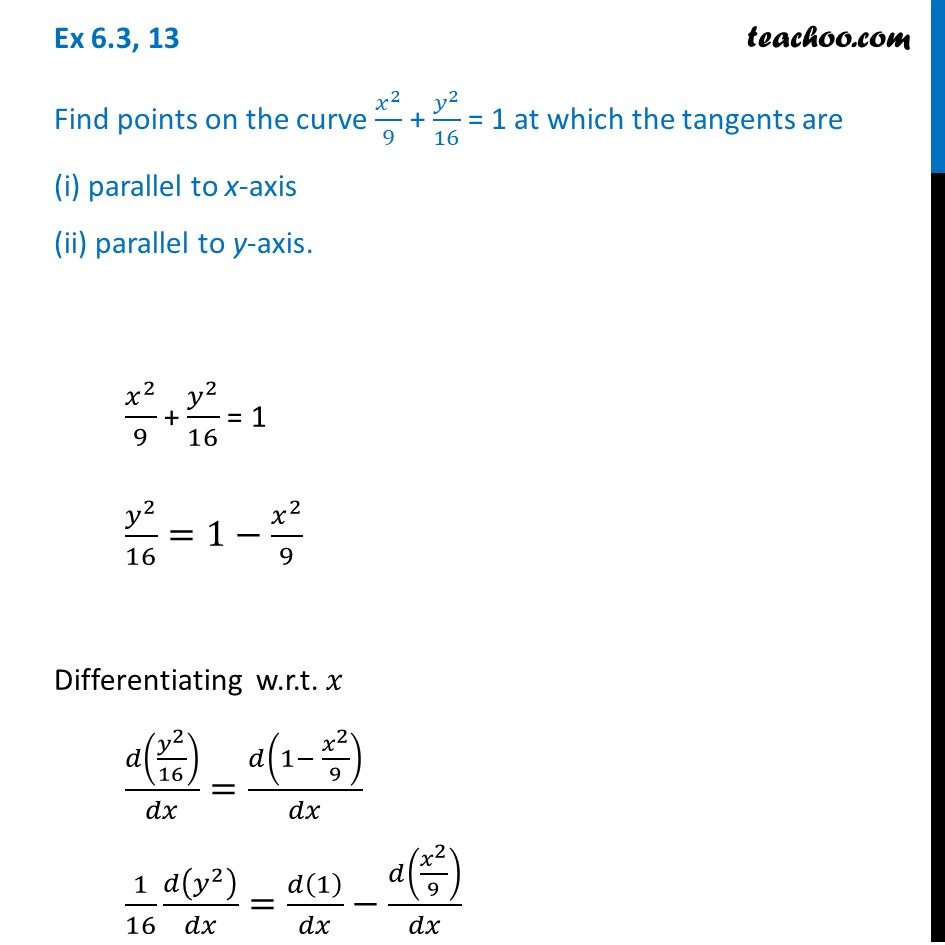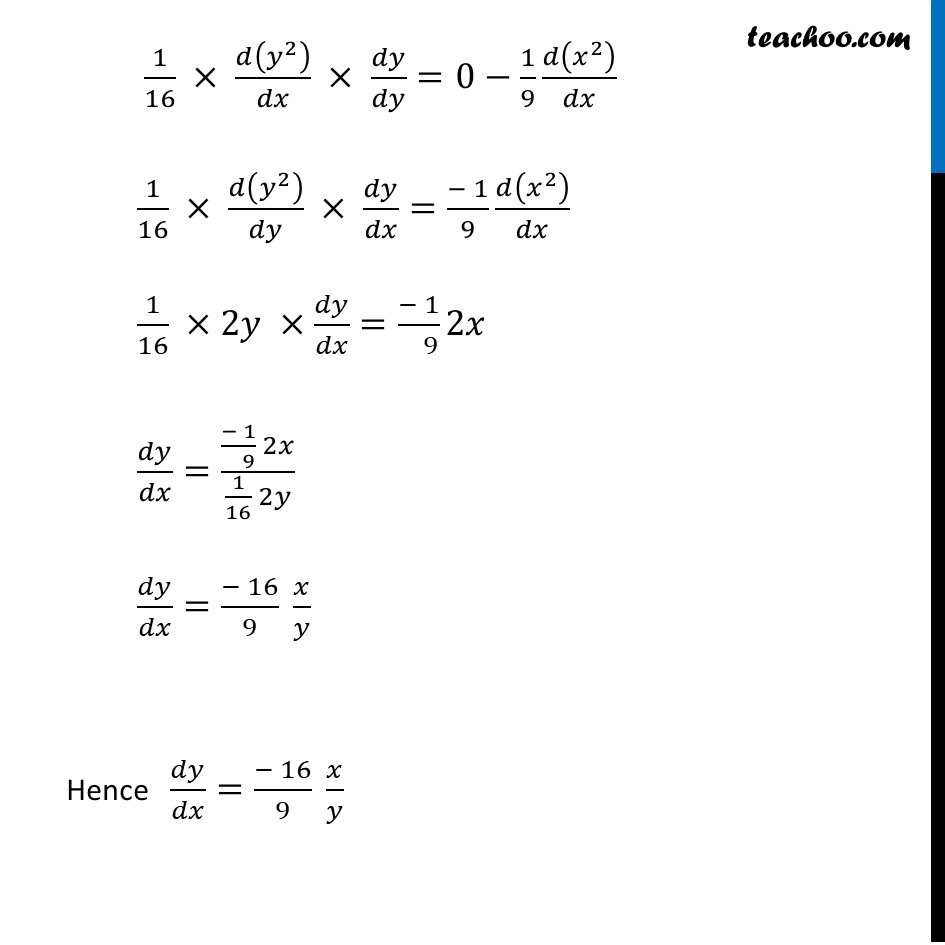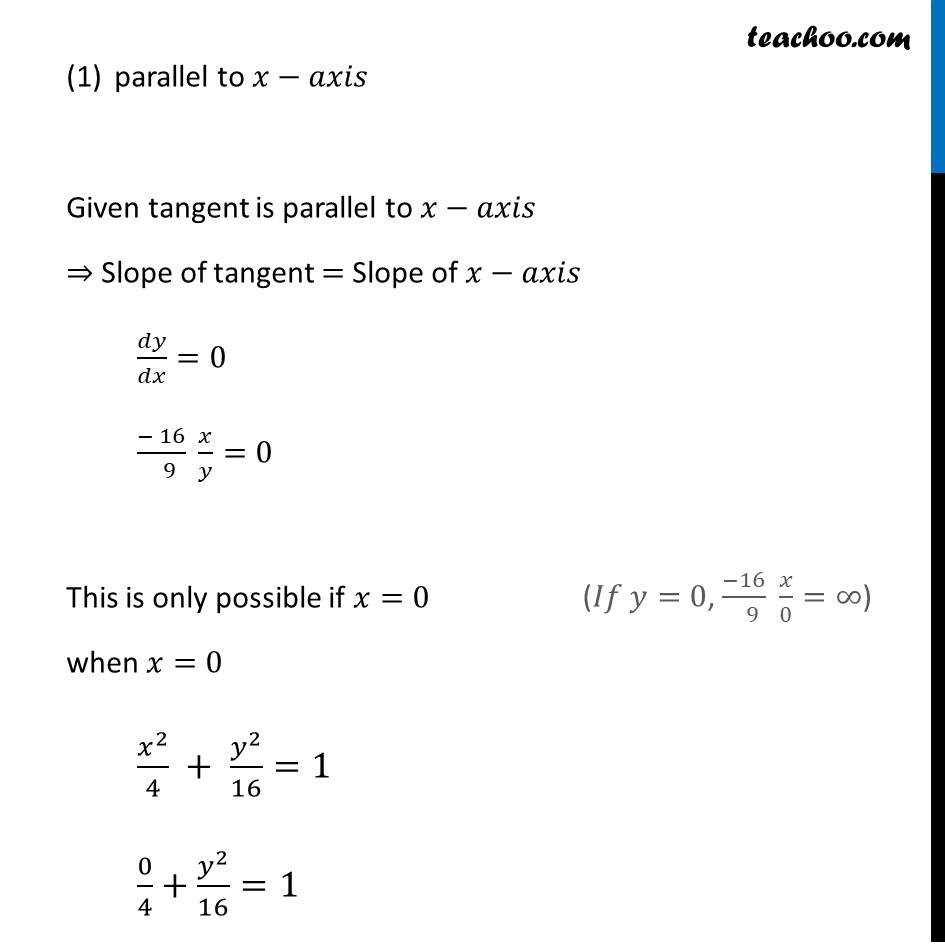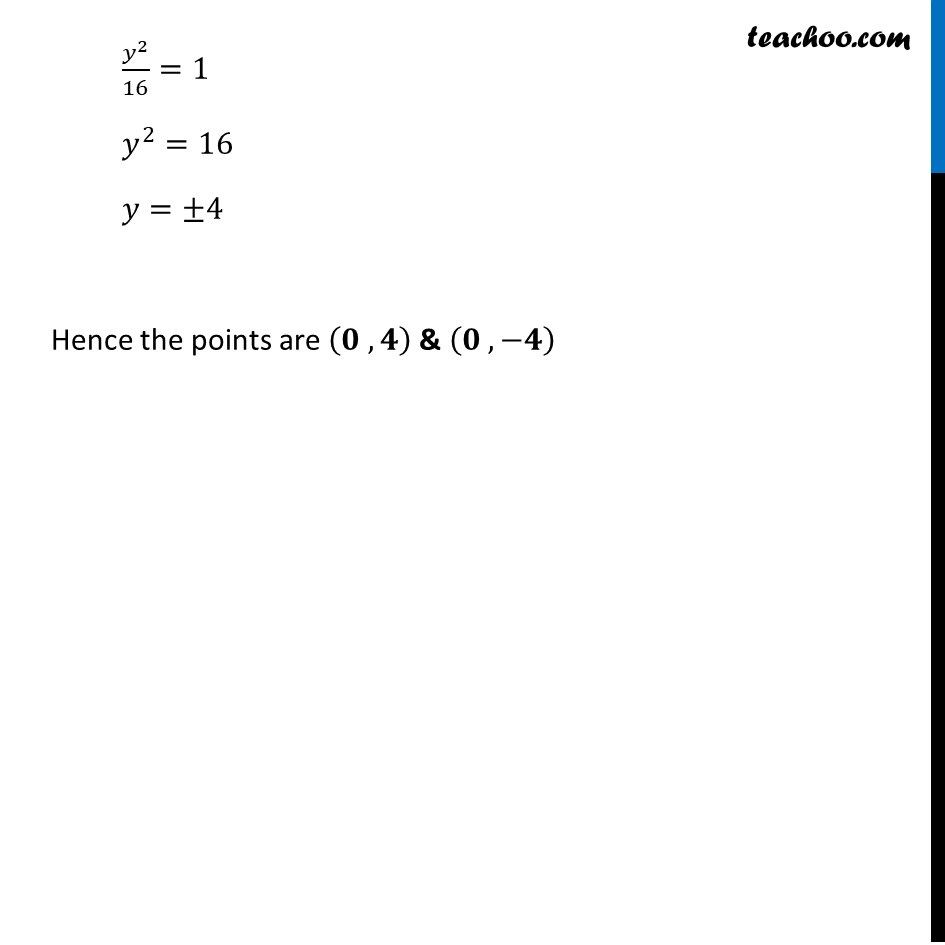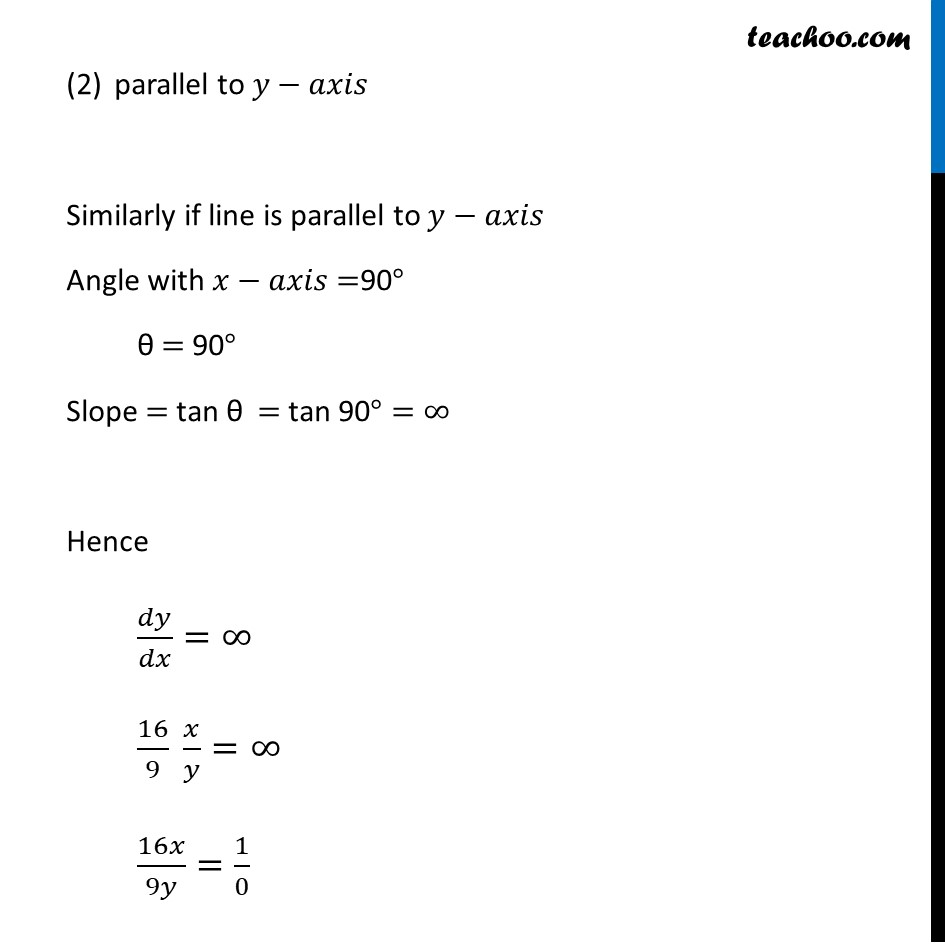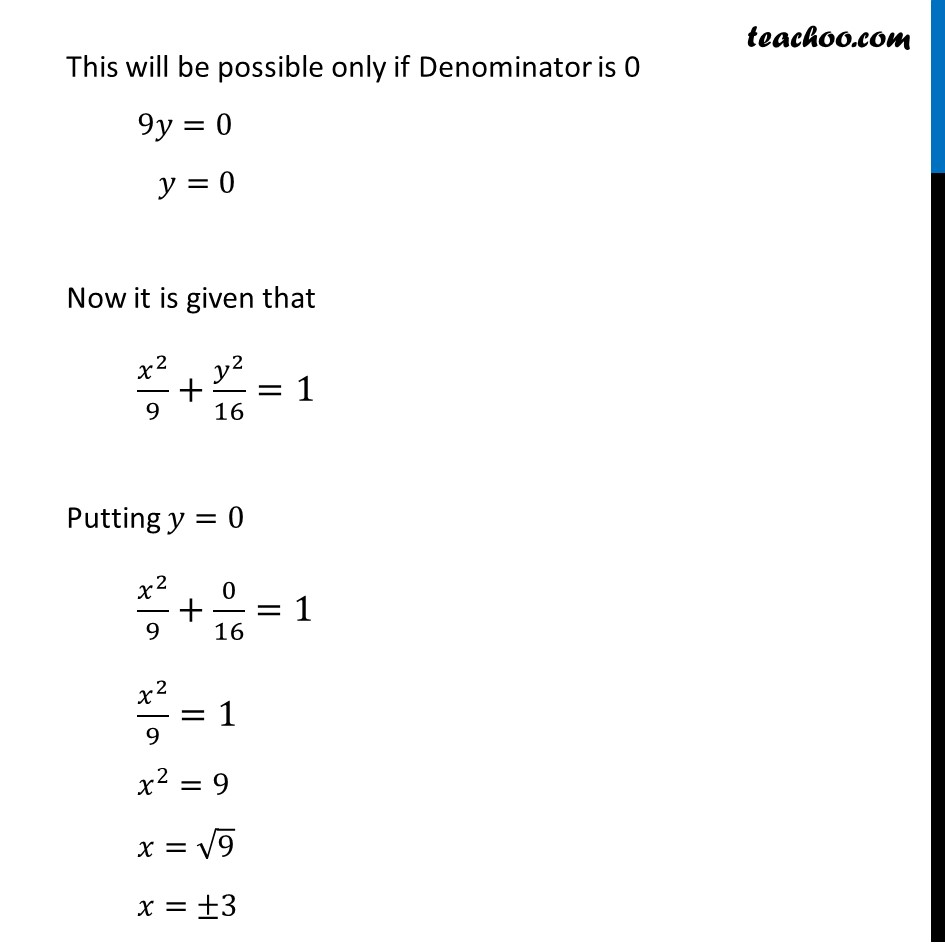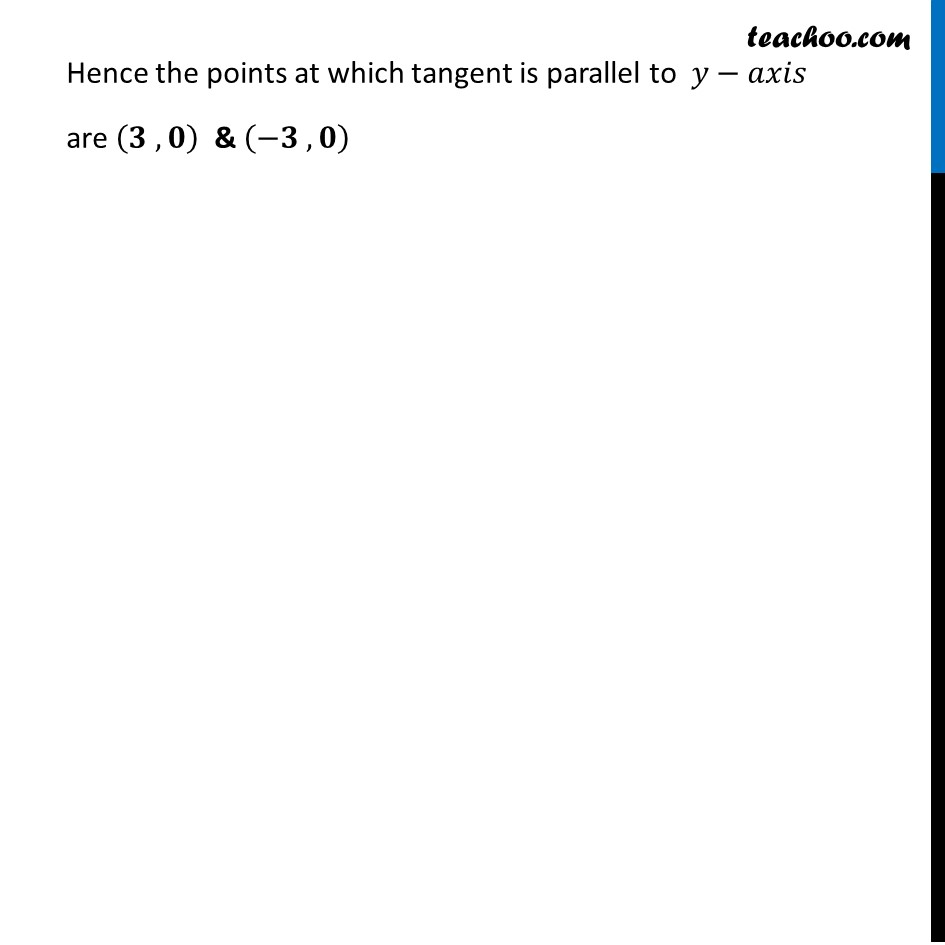Introducing your new favourite teacher - Teachoo Black, at only ₹83 per month

### Transcript

Ex 6.3, 13 Find points on the curve 𝑥^2/9 + 𝑦^2/16 = 1 at which the tangents are (i) parallel to x-axis (ii) parallel to y-axis. 𝑥^2/9 + 𝑦^2/16 = 1 𝑦^2/16=1−𝑥^2/9 Differentiating w.r.t. 𝑥 𝑑(𝑦^2/16)/𝑑𝑥=𝑑(1− 𝑥^2/9)/𝑑𝑥 1/16 𝑑(𝑦^2 )/𝑑𝑥=𝑑(1)/𝑑𝑥−𝑑(𝑥^2/9)/𝑑𝑥 1/16 × 𝑑(𝑦^2 )/𝑑𝑥 × 𝑑𝑦/𝑑𝑦=0−1/9 𝑑(𝑥^2 )/𝑑𝑥 1/16 × 𝑑(𝑦^2 )/𝑑𝑦 × 𝑑𝑦/𝑑𝑥=(− 1)/9 𝑑(𝑥^2 )/𝑑𝑥 1/16 ×2𝑦 ×𝑑𝑦/𝑑𝑥=(− 1)/( 9) 2𝑥 𝑑𝑦/𝑑𝑥=((− 1)/( 9) 2𝑥)/(1/16 2𝑦) 𝑑𝑦/𝑑𝑥=(− 16)/9 𝑥/𝑦 Hence 𝑑𝑦/𝑑𝑥=(− 16)/9 𝑥/𝑦 parallel to 𝑥−𝑎𝑥𝑖𝑠 Given tangent is parallel to 𝑥−𝑎𝑥𝑖𝑠 ⇒ Slope of tangent = Slope of 𝑥−𝑎𝑥𝑖𝑠 𝑑𝑦/𝑑𝑥=0 (− 16)/( 9) 𝑥/𝑦=0 This is only possible if 𝑥=0 when 𝑥=0 𝑥^2/4 + 𝑦^2/16=1 0/4+𝑦^2/16=1 (𝐼𝑓 𝑦=0, (−16)/( 9) 𝑥/0=∞) 𝑦^2/16=1 𝑦^2=16 𝑦=±4 Hence the points are (𝟎 , 𝟒) & (𝟎 , −𝟒) parallel to 𝑦−𝑎𝑥𝑖𝑠 Similarly if line is parallel to 𝑦−𝑎𝑥𝑖𝑠 Angle with 𝑥−𝑎𝑥𝑖𝑠 =90° θ = 90° Slope = tan θ = tan 90°=∞ Hence 𝑑𝑦/𝑑𝑥=∞ 16/9 𝑥/𝑦=∞ 16𝑥/9𝑦=1/0 This will be possible only if Denominator is 0 9𝑦=0 𝑦=0 Now it is given that 𝑥^2/9+𝑦^2/16=1 Putting 𝑦=0 𝑥^2/9+0/16=1 𝑥^2/9=1 𝑥^2=9 𝑥=√9 𝑥=±3 Hence the points at which tangent is parallel to 𝑦−𝑎𝑥𝑖𝑠 are (𝟑 , 𝟎) & (−𝟑 , 𝟎)